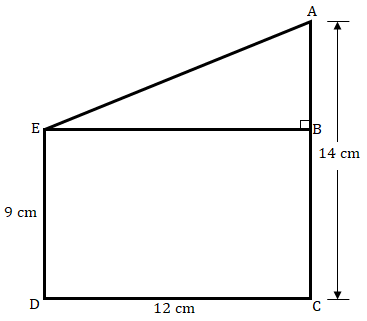"
">

# Two poles of height 9 m and 14 m stand on a plane ground. If the distance between their feet is 12 m, find the distance between their tops."

Given:

Two poles of height 9 m and 14 m stand on a plane ground.

The distance between their feet is 12 m.

To do:

We have to find the distance between their tops.

Solution:

From the figure,

$AB = AC – BC$

$AB = (14 – 9)\ m = 5\ m$

$EB = DC = 12\ m$.

In $∆ABE$,

By Pythagoras theorem,

$AE^2 = AB^2 + BE^2$

$AE^2 = 5^2 + 12^2$

$AE^2 = 25 + 144$

$AE^2 = 169$

$AE = \sqrt{169} = 13\ m$

Therefore, the distance between their tops is $13\ m$.

Updated on: 10-Oct-2022

38 Views